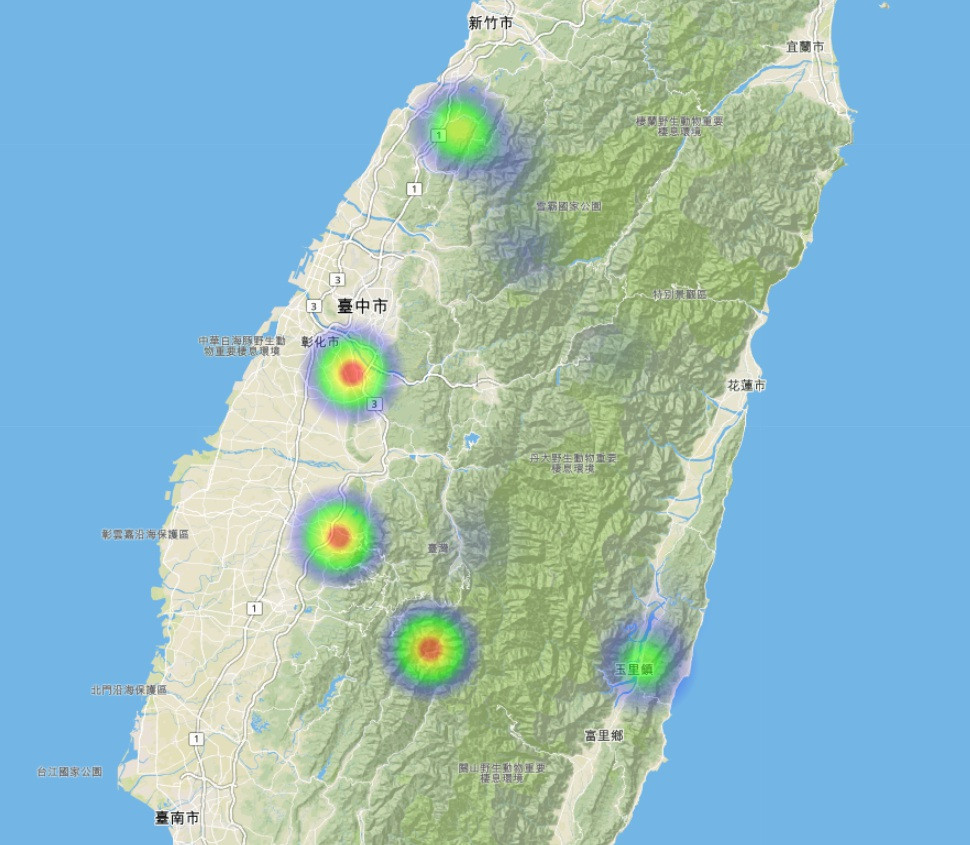#DAY 23
0
Modern Web

## [8-2] heatmap.js 熱區－ 以Leaflet地圖實作

[8-1] 展點多到爆？那就試試看 Leaflet MarkerCluster吧！

John Snow的貢獻也為流行病學及空間地理學有重要的開端。

# heatmap.js

↓ 透過npm下載heatmap.js，不愛用npm的人可以去githubheatmap.js文件中下載

``````    npm install heatmap.js
``````

↓ 下載完後，引入heatmap.js

``````    <script src="node_modules/heatmap.js/build/heatmap.min.js"></script>
``````

↓建立一個用以存放heatmap canvas的div

``````    <div class="heatmap"></div>
``````

↓ 起手式：h337.create()

``````        const heatmapInstance = h337.create({
container: document.querySelector('.heatmap'),  //存放heatmap的div
});
``````

↓ 然後我們利用昨天寫的亂數產生器來產生範例點座標

``````        function random(min, max) {
return Math.random() * (max - min) + min;
}
``````

↓ 相較於昨天，陣列中除了x座標及y座標外，熱區地圖還需要每個點的數值大小，以決定熱區的核密度，也就是點資料聚集的程度。

``````        let arr = [];
function CreatePoint(count) {
for (let i = 0; i < count; i++) {
let x = Math.floor(random(0, window.innerWidth));
let y = Math.floor(random(0, window.innerHeight));
let value = Math.floor(random(0, 100));

arr.push({ x: x, y: y, value: value });
}
}
``````

`HTML5 Canvas是以左上角為中心，x向右為正，y向下為正。因此x座標範圍設定從0~畫面寬度，y從0~畫面高度。`

↓ 產生點

``````        CreatePoint(1500);
const data = {  // 熱區繪製的資料格式
max: 100,
data: arr
};
console.log(data)
``````

↓ console亂數產生的點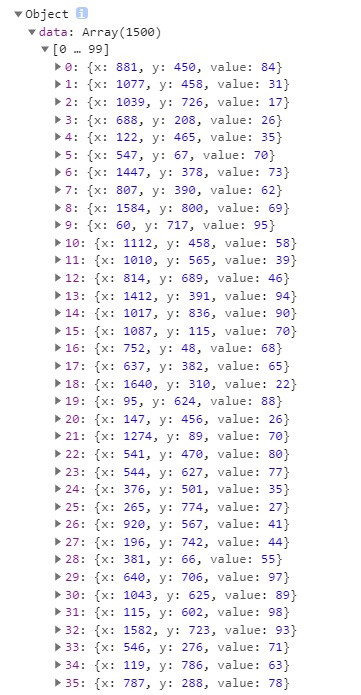↓ 呼叫熱區圖

``````        heatmapInstance.setData(data);
``````

↓ 結果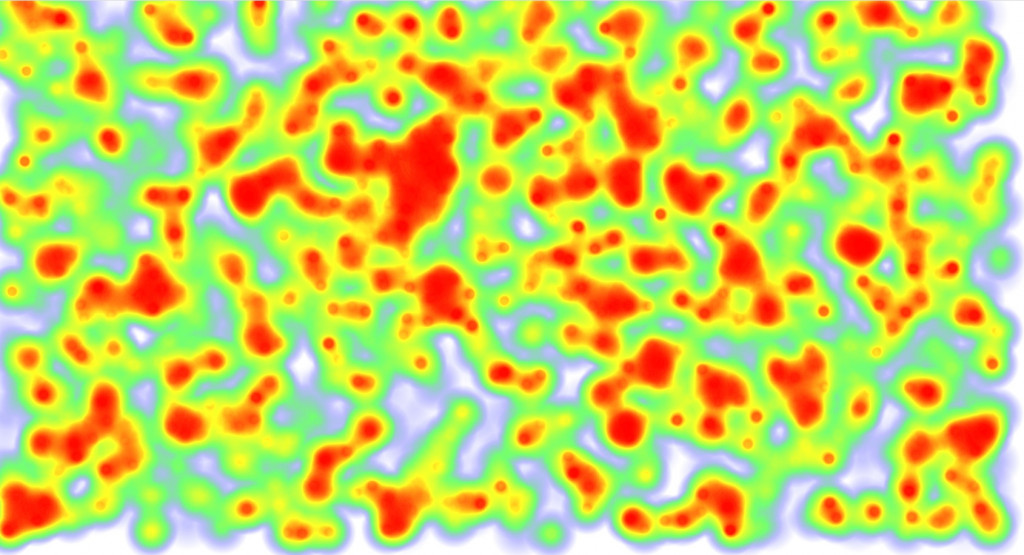# 熱區圖設定及滑鼠事件

• ## 打造自己的熱區圖

↓ 熱區圖可以設定核密度半徑、背景顏色、梯度等等，來打造自己的熱區圖吧！

``````        const heatmapInstance = h337.create({
container: document.querySelector('.heatmap'),
backgroundColor: 'rgba(0,0,0,.75)',
//radius: 30,
gradient: {
// enter n keys between 0 and 1 here
// for gradient color customization
'.5': 'blue',
'.8': 'red',
'.95': 'yellow'
},
maxOpacity: .9,
minOpacity: .3
});
``````

h337的熱區圖設定

• container： 存放canvas的容器
• backgroundColor： 背景顏色
• radius： 核密度半徑
• gradient：梯度及梯度顏色
• opacity： 預設透明度
• minOpacity： 最小數值透明度
• maxOpacity： 最大數值的透明度
• xField： x座標欄位名稱，預設為"x"
• yField： y座標欄位名稱，預設為"y"
• valueField ： 數值欄位名稱，預設為"value"
• onExtremaChange： 極值更新的change事件，用於動態算級距時改變圖例使用
• blur： 模糊度，越高則邊界越順暢，預設為0.85

↓ 結果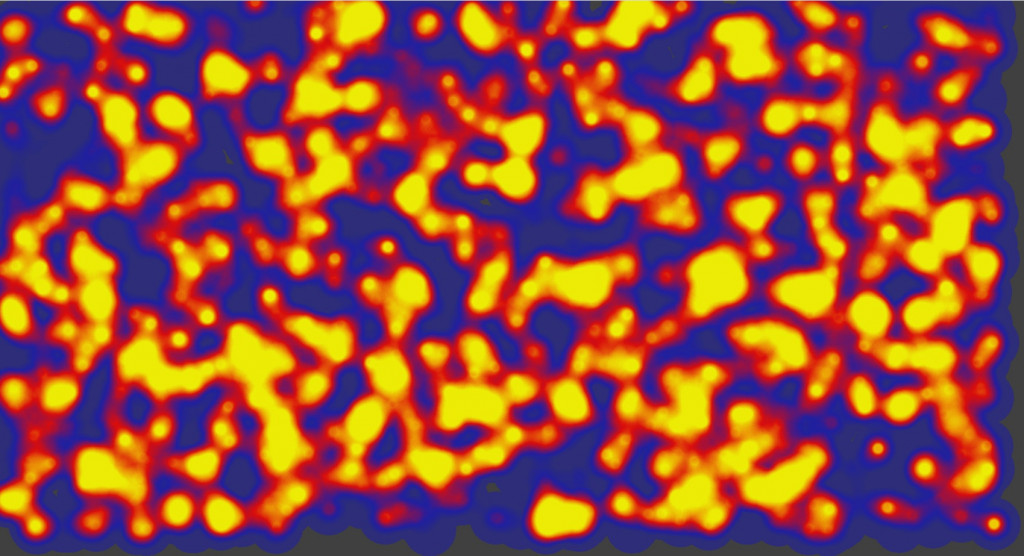• ## 滑鼠事件

↓ 可以綁定dom元素的滑鼠事件，並且把滑鼠位置塞值給熱區圖物件。

``````        document.querySelector('.heatmap').onmousemove = function (e) {
heatmapInstance.addData({
x: e.layerX,
y: e.layerY,
value: 1
});
};
``````

↓ 可以用滑鼠畫的熱區畫布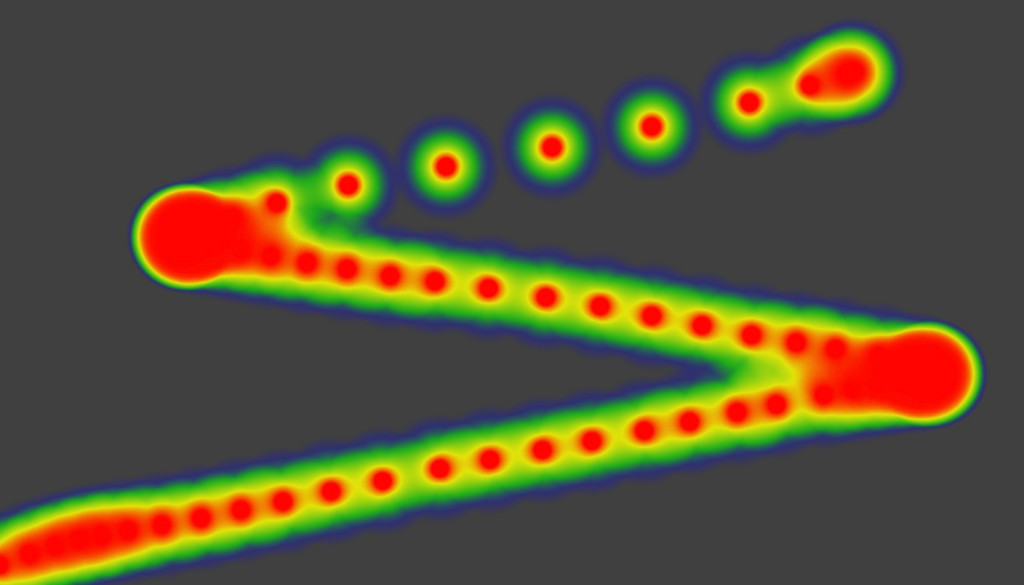# Leaflet熱區地圖

• ## 前置作業

↓ 引入heatmap.js外，還要引入leaflet-heatmap.js。

``````<script src="node_modules/heatmap.js/build/heatmap.min.js"></script>
<script src="node_modules/heatmap.js/plugins/leaflet-heatmap/leaflet-heatmap.js"></script>
``````

↓ 存放地圖的div

``````    <div id="lmap"></div>
``````

↓ 讓地圖滿板

``````    <style>
html,
body {
padding: 0;
margin: 0;
height: 100%;
}

#lmap {
height: 100%;
}
</style>
``````

↓ 初始化地圖

``````        const LMap = L.map(document.getElementById('lmap'), {
center: [23.5, 121],
zoom: 7,
crs: L.CRS.EPSG3857,
});
L.tileLayer('https://api.tiles.mapbox.com/v4/{id}/{z}/{x}/{y}.png?access_token=pk.eyJ1IjoibWFwYm94IiwiYSI6ImNpejY4NXVycTA2emYycXBndHRqcmZ3N3gifQ.rJcFIG214AriISLbB6B5aw', {
maxZoom: 18,
id: 'mapbox.streets'
}).addTo(LMap);
``````

↓ 結果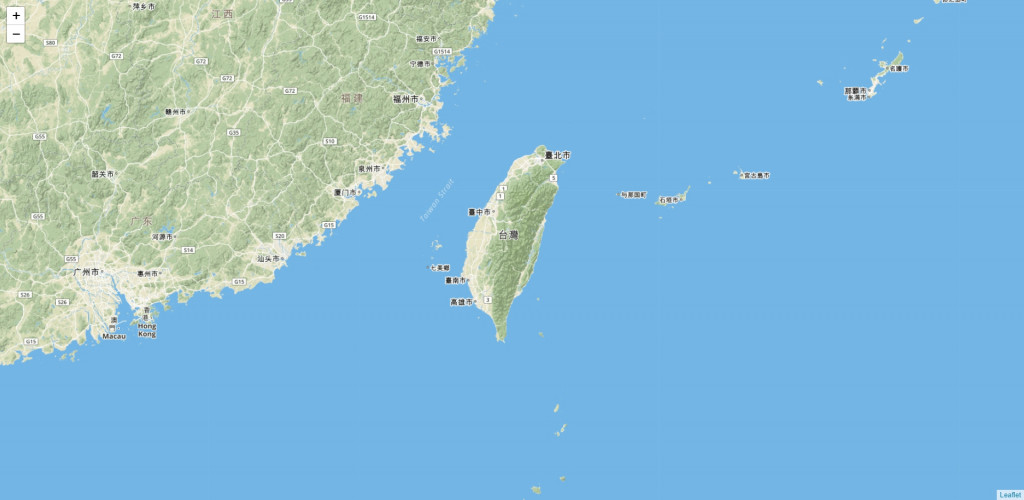↓ 這次我們亂數產生點，給定經度範圍及緯度範圍。

``````        let arr = [];
function CreatePoint(count) {
for (let i = 0; i < count; i++) {
let longitude = random(120.5, 121.4);
let latitude = random(23, 24.6);
let value = Math.floor(random(0, 100));

arr.push({ x: longitude, y: latitude, value: value });
}
}
function random(min, max) {
return Math.random() * (max - min) + min;
}
CreatePoint(100);
``````
• ## 熱區地圖

↓ 熱區地圖設定

``````        const option = {
"scaleRadius": false,
"radius": 50,
"useLocalExtrema": true,
latField: 'y',
lngField: 'x',
valueField: 'value',
"maxOpacity": .5
};
``````

`leaflet-heatmap.js使用HeatmapOverlay物件，`
`比起heatmap.js的h337物件，多了三種設定`

• radius： 核密度半徑
• scaleRadius： 如果為true會根據地圖縮放層級來測量；如果為false，不論縮放層級為何均以核密度半徑(單位為px)來計算。
• useLocalExtrema：當前極值或定極值。如果為false，使用全域極值，也就是固定的最大最小極距；如果為true，則是使用當前最大數值及最小數值作為級距。

↓ 呼叫熱區地圖

``````        const heatmapLayer = new HeatmapOverlay(option);
``````

↓ 設定熱區地圖物件的資料

``````        const testData = {
max: 100,
data: arr
};
heatmapLayer.setData(testData);
``````

↓ 把熱區地圖加入Leaflet圖台

``````        heatmapLayer.addTo(LMap);
``````
• ## 結果比較

`核密度半徑`

↓ radius 30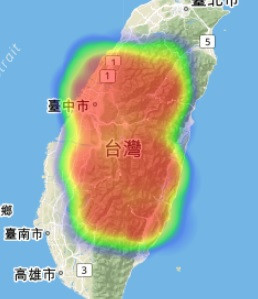↓ 放大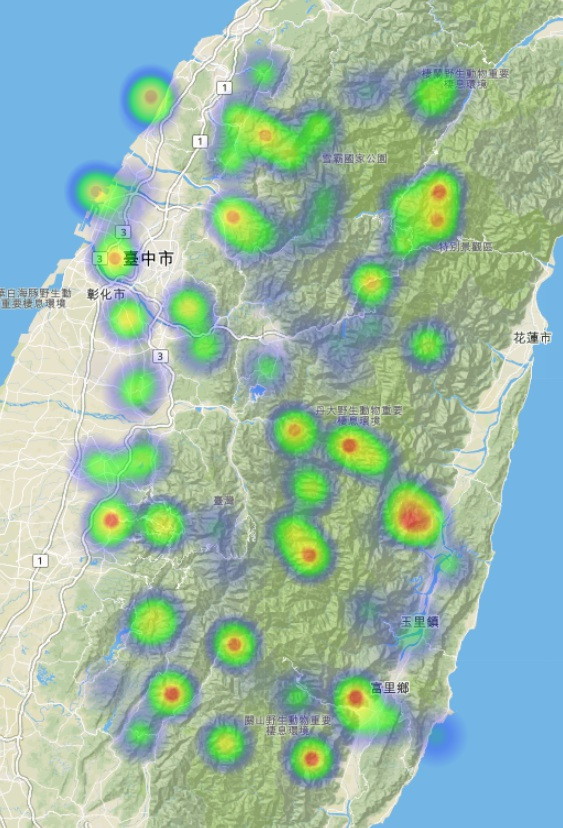↓ radius 50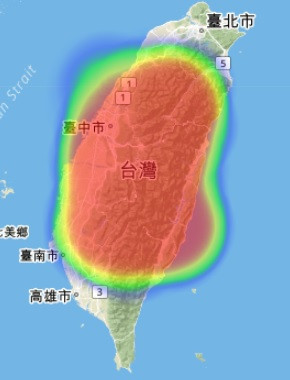↓ 放大`當前極值及定極值`
useLocalExtrema：當前極值或定極值。如果為false，使用全域極值，也就是固定的最大最小極距；如果為true，則是使用當前最大數值及最小數值作為級距。

↓ useLocalExtrema: false 定極值(全域極值)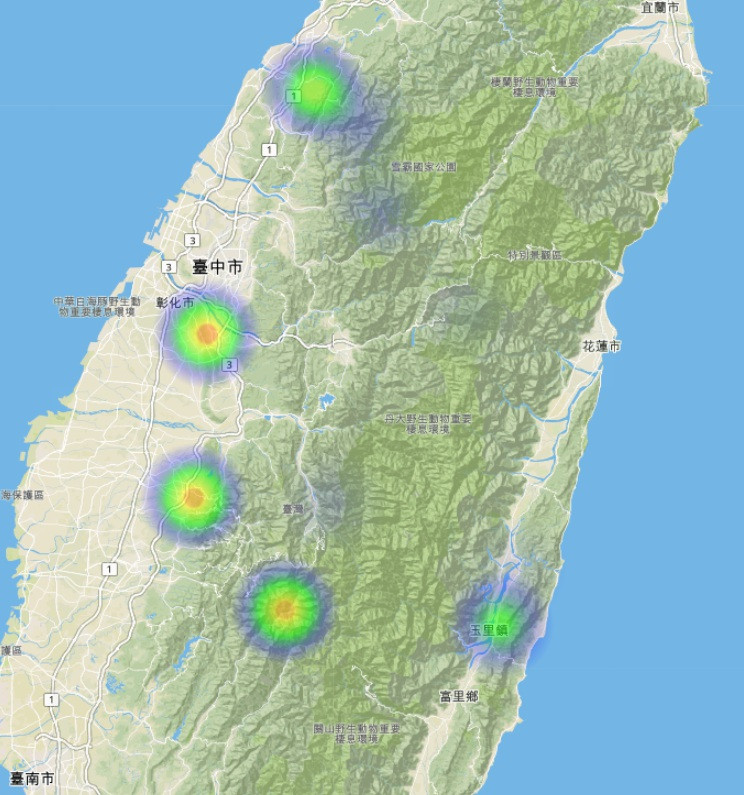↓ useLocalExtrema: true 當前極值